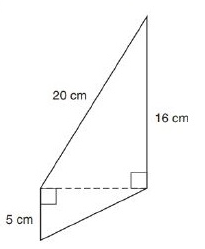Chapter 8.2, Problem 8E### Elementary Geometry for College St...

6th Edition
Daniel C. Alexander + 1 other
ISBN: 9781285195698

#### Solutions

Chapter
Section### Elementary Geometry for College St...

6th Edition
Daniel C. Alexander + 1 other
ISBN: 9781285195698
Textbook Problem
5 views

# In Exercises 1 to 8, find the perimeter of each polygon.To determine

To Find:

Explanation

Formula Used:

The perimeter of a polygon is the sum of the lengths of all sides of the polygon.

Pythagorean theorem for the right angle triangle ABC for the hypotenuse AC,

AC2=AB2+BC2.

Calculation:

Let the vertices of the quadrilateral be A, B, C and D.

Both the ABC and ACD are right angled triangle. Therefore, both satisfied Pythagorean theorem.

Consider the right triangle ACD for the hypotenuse AD,

AC2=20</

### Still sussing out bartleby?

Check out a sample textbook solution.

See a sample solution

#### The Solution to Your Study Problems

Bartleby provides explanations to thousands of textbook problems written by our experts, many with advanced degrees!

Get Started

#### In Problems 29-36, find the particular solution to each differential equation.

Mathematical Applications for the Management, Life, and Social Sciences

#### Describe mathematical modeling in your own words.

Applied Calculus for the Managerial, Life, and Social Sciences: A Brief Approach

#### True or False: converges.

Study Guide for Stewart's Single Variable Calculus: Early Transcendentals, 8th